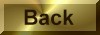Practice Problem 6

Calculate the molecular weight of sulfur if 35.5 grams of sulfur dissolve in 100.0 grams of CS2 to produce a solution that has a boiling point of 49.48oC.

Solution

The relationship between the boiling point of the solution and the molecular weight of sulfur is not immediately obvious. We therefore start by asking: What do we know about this problem?

We know the boiling point of the solution, so we might start by looking up the boiling point of the pure solvent in order to calculate the change in the boiling point that occurs when the sulfur is dissolved in CS2.TBP = 49.48oC - 46.23oC = 3.25oC

We also know that the change in the boiling point is proportional to the molality of the solution.TBP = kbm

Since we know the change in the boiling point (TBP) and the boiling point elevation constant for the solvent (kb) can be looked up in a table, we might decide to calculate the molality of the solution at this point.

 m =TBP = 3.25oC = 1.38 m kb 2.35oC/m

In the search for the solution to a problem, it is useful periodically to consider what we have achieved so far. At this point, we know the molality of the solution and the mass of the solvent used to prepare the solution. We can therefore calculate the number of moles of sulfur present in 100.0 grams of carbon disulfide.

 1.38 mol sulfur x 100.0 g CS2 = 0.138 mol sulfur 1000 g CS2

We now know the number of moles of sulfur in this solution and the mass of the sulfur. We can therefore calculate the number of grams per mole of sulfur.

 35.5 g = 257 g/mol 0.138 mol

The only way to explain this molecular weight is to assume that each sulfur molecule contains eight sulfur atoms.

 257 g/mol = 8 32 g/mol

Elemental sulfur therefore behaves as if it contains S8 molecules.